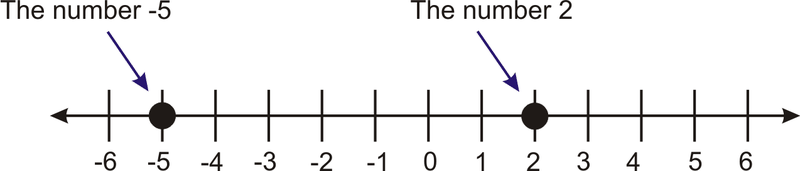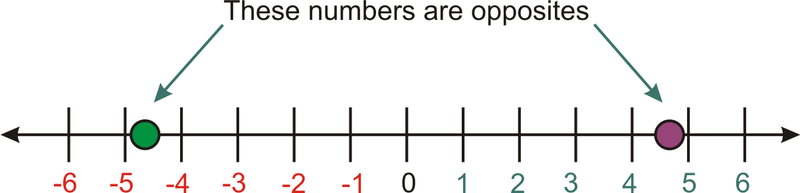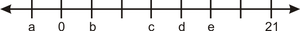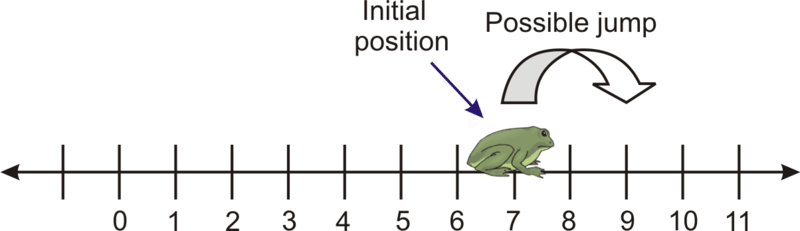# 1.6.6: Additive Inverses and Absolute Value

•• Contributed by CK12
• CK12

## Additive Inverses and Absolute Values

Suppose that you are creating a budget and that you have expenses of $2500 per month. How much money would you have to bring in each month in order to break even? Would the expenses be thought of as a positive or negative number? What would be the additive inverse of the expenses? What would be the absolute value? ### Adding Inverses and Absolute Values #### Graphing and Comparing Integers More specific than the rational numbers are the integers. Integers are whole numbers and their negatives. When comparing integers, you will use the math verbs such as less than, greater than, approximately equal to, and equal to. To graph an integer on a number line, place a dot above the number you want to represent. Compare the following integers: 2 and -5. First, we will plot the two numbers on a number line.Figure 6.6.1 We can compare integers by noting which is the greatest and which is the least. The greatest number is farthest to the right, and the least is farthest to the left. In the diagram above, we can see that 2 is farther to the right on the number line than -5, so we say that 2 is greater than -5. We use the symbol > to mean “greater than.” Therefore, 2 > -5. #### Numbers and Their Opposites Every real number, including integers, has an opposite, which represents the same distance from zero but in the other direction.Figure 6.6.2 A special situation arises when adding a number to its opposite. The sum is zero. This is summarized in the following property: • The Additive Inverse Property: For any real number a, a+(-a)=0 We see that -a is the additive inverse, or opposite, of a. Find the opposite number of the following numbers: 1. -5 The opposite number of -5 is 5. Using the Additive Inverse Property: -5+(5)=0 1. 1/2 The opposite number of 1/2 is -1/2. The Additive Inverse Property shows us that they are opposites: 12+(-12)=0 1. 5.1 The opposite number of 5.1 is -5.1. The Additive Inverse Property gives: 5.1+(-5.1)=0 #### Absolute Value Absolute value represents the distance from zero when graphed on a number line. For example, the number 7 is 7 units away from zero. The number -7 is also 7 units away from zero. The absolute value of a number is the distance it is from zero, so the absolute value of 7 and the absolute value of -7 are both 7. A number and its additive inverse are always the same distance from zero, and so they have the same absolute value. We write the absolute value of -7 like this: |-7|. We read the expression |x| like this: “the absolute value of x.” • Treat absolute value expressions like parentheses. If there is an operation inside the absolute value symbols, evaluate that operation first. • The absolute value of a number or an expression is always positive or zero. It cannot be negative. With absolute value, we are only interested in how far a number is from zero, not the direction. Evaluate the following absolute value expressions: 1. |5+4| |5+4|=|9|=9 1. 3−|4−9| 3−|4−9|=3−|−5|=3−5=−2 1. |-5−11| |-5−11|=|-16|=16 1. -|7−22| -|7−22|=-|-15|=-(15)=-15 ### Examples Example $$\PageIndex{1}$$ Earlier, you were told that you should create a budget. You have expenses of$2500 per month. How much money would you have to bring in each month in order to break even? Would the expenses be thought of as a positive or negative number? What would be the additive inverse of the expenses? What would be the absolute value?

Solution

The word expenses indicates that you are spending $2500 a month. Therefore, the expenses should be thought of a negative number because that is the amount of money that is leaving your bank account. With the expenses, you have -$2500 in your bank account and to break even, you would need to make enough money to have $0 in your bank account. In other words, you need to find the additive inverse. The additive inverse of -2500 is 2500. So you would need to make$2500 to break even each month. The absolute value of both the expenses and the amount you need to earn is 2500.

Example $$\PageIndex{1}$$

What is the opposite of x−1?

Solution

The opposite of x−1 is -(x−1). We can use the Additive Inverse Property to prove it:

Since -(x−1)=-x−1, we can see that

(x−1)+(-(x−1))=(x−1)+(-x−1)=0.

Example $$\PageIndex{1}$$

Evaluate the following:

|3−4|−2

Solution

|3−4|−2=|-1|−2=1−2=-1

Example $$\PageIndex{1}$$

Evaluate the following

|5−7.5|+3

Solution

|5−7.5|+3=|-2.5|+3=2.5+3=5.5

### Review

1. Define absolute value.
2. Give an example of a real number that is not an integer.
3. The tick-marks on the number line represent evenly spaced integers. Find the values of a,b,c,d,and e:In 4–9, find the opposite of each of the following.

1. 1.001
2. –9.345
3. (16 – 45)
4. (5 – 11)
5. (x+y)
6. (x−y)

In 10–19, simplify.

1. |-98.4|
2. |123.567|
3. -|16−98|
4. 11−|-4|
5. |4−9|−|-5|
6. |-5−11|
7. 7−|22−15−19|
8. -|-7|
9. |-2−88|−|88+2|
10. |-5−99|+-|16−7|

In 20–25, compare the two real numbers.

1. 8 and 7.99999
2. -4.25 and -174
3. 65 and -1
4. 10 units left of zero and 9 units right of zero
5. A frog is sitting perfectly on top of number 7 on a number line. The frog jumps randomly to the left or right, but always jumps a distance of exactly 2. Describe the set of numbers that the frog may land on, and list all the possibilities for the frog’s position after exactly 5 jumps.6. Will a real number always have an additive inverse? Explain your reasoning.

### Vocabulary

Term Definition
absolute value The absolute value of a number represents the distance from zero when graphed on a number line.
Additive Inverse Property For any real number a, a+(−a)=0. We see that −a is the additive inverse, or opposite, of a.
integers An integer is a real number which is a whole number or the negative (opposite) of a whole number.
opposite number An opposite number of a number a represents the same distance from zero as a, but in the other direction.

Video:

PLIX: Play, Learn, Interact, eXplore: Opposites and Absolute Values: Painting a Fence

Activity: Absolute Value Discussion Questions

Study Aid: Number Lines Study Guide

Practice: Additive inverses and Absolute Values

Real World Application: Absolute Value